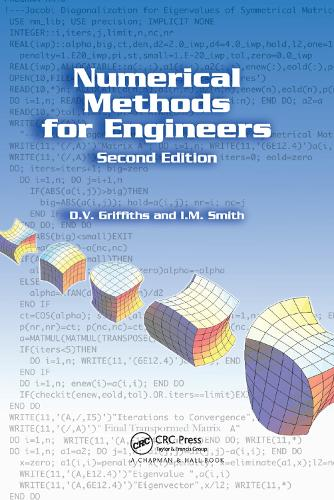•# Numerical Methods for Engineers (Paperback)

(author), (author)
£54.99
Paperback 492 Pages / Published: 19/09/2019
• We can order this

Usually dispatched within 3 weeks

Although pseudocodes, Mathematica ®, and MATLAB ® illustrate how algorithms work, designers of engineering systems write the vast majority of large computer programs in the Fortran language. Using Fortran 95 to solve a range of practical engineering problems, Numerical Methods for Engineers, Second Edition provides an introduction to numerical methods, incorporating theory with concrete computing exercises and programmed examples of the techniques presented.

Covering a wide range of numerical applications that have immediate relevancy for engineers, the book describes forty-nine programs in Fortran 95. Many of the programs discussed use a sub-program library called nm_lib that holds twenty-three subroutines and functions. In addition, there is a precision module that controls the precision of calculations.

Well-respected in their field, the authors discuss a variety of numerical topics related to engineering. Some of the chapter features include...
The numerical solution of sets of linear algebraic equations
Roots of single nonlinear equations and sets of nonlinear equations
Numerical quadrature, or numerical evaluation of integrals
An introduction to the solution of partial differential equations using finite difference and finite element approaches

Describing concise programs that are constructed using sub-programs wherever possible, this book presents many different contexts of numerical analysis, forming an excellent introduction to more comprehensive subroutine libraries such as the numerical algorithm group (NAG).

Publisher: Taylor & Francis Ltd
ISBN: 9780367390662
Number of pages: 492
Weight: 2000 g
Dimensions: 234 x 156 mm
Edition: 2nd New edition